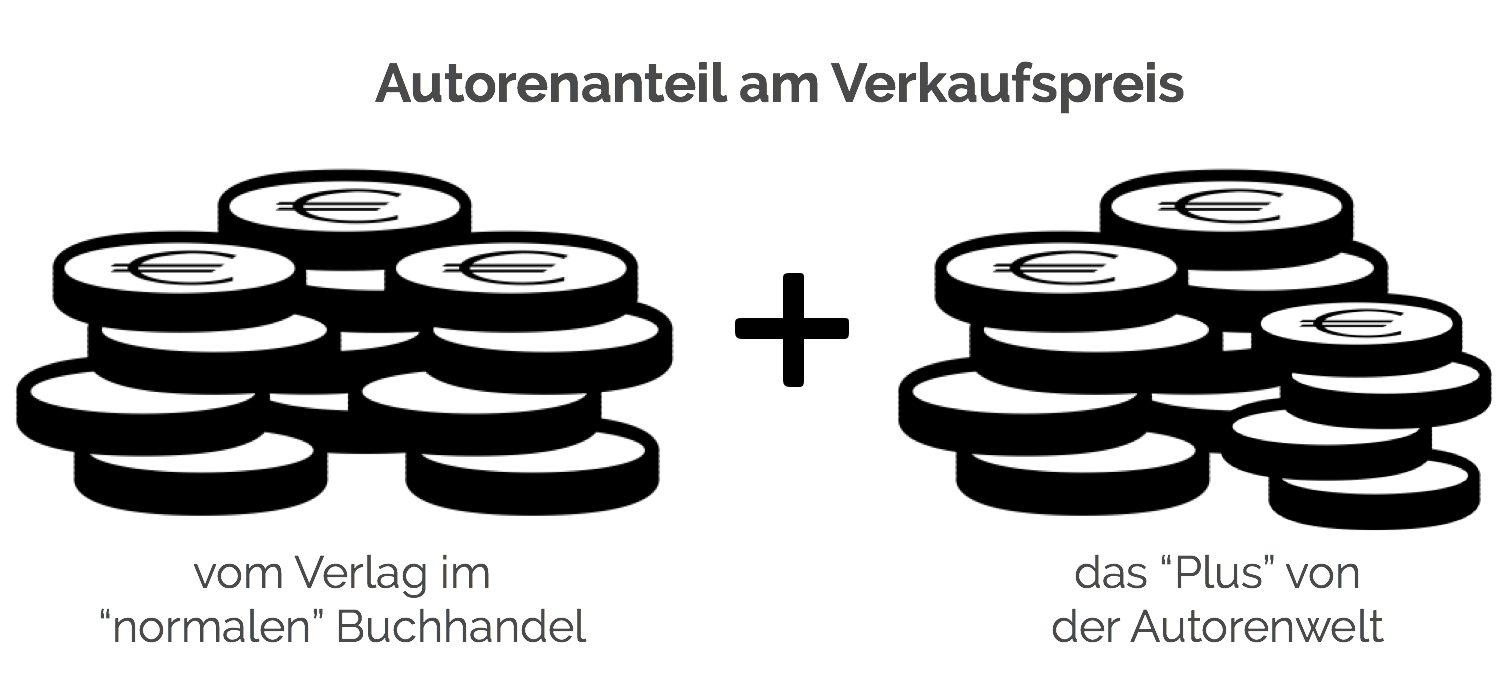# The q -state Potts Model: Partition functions and their zeros in the complex temperature- and q-plane

##### von Hubert Klüpfel
Taschenbuch - 9783656297390
33,99 €
• Versandkostenfrei
• Hinweis: Print on Demand. Lieferbar innerhalb von 7 bis 10 Tagen
• inkl. MwSt. & Versandkosten (innerhalb Deutschlands)
• Hinweis: Print on Demand. Lieferbar innerhalb von 7 bis 10 Tagen
• inkl. MwSt. & Versandkosten (innerhalb Deutschlands)

## Autorenfreundlich Bücher kaufen?!## Beschreibung

Master's Thesis from the year 1999 in the subject Physics - Theoretical Physics, grade: 1,0, State University of New York at Stony Brook (Institute for Theoretical Physics), course: -, language: English, abstract: In this thesis results on the Partition function ZG(T, q) for the q-state Potts-Model on finite polygonal lattices G are presented. These are polynomials in a = e- J and q. The first step is to calculate all the coefficients of ZG(a, q) using a transfer matrix method. The only points of non-analyticity are the zeros of the partition function; in the thermodynamic limit the complex temperature zeros form a continuous curve B via coalescence. This is the locus where the free energy is non-analytic. The zeros of Z(a, q) in the complex q-plane for finite a and in the complex a-plane for integer and non-integer values of q are plotted. For a = 0 the partition function reduces to the chromatic polynomial PG(q) of the graph and the zeros are called chromatic zeros. The behavior of those zeros as a increases from zero is investigated. As for complex temperature the zeros in q form a continuous curve in the thermodynamic limit. This is the locus where the limiting function WG(q) = limn¿8 PG(q)1/n is non-analytic. WG(q) is the ground state degeneracy and is connected to the ground state entropy via S0(G, q) = kB ln(WG(q)). Thus the characteristics of the zeros of q and a for finite lattices help to understand the properties of the model in the thermodynamic limit.

## Details

 Verlag GRIN Publishing Ersterscheinung März 2014 Maße 210 mm x 148 mm x 9 mm Gewicht 195 Gramm Format Taschenbuch ISBN-13 9783656297390 Auflage 1. Auflage Seiten 128

## Schlagwörter

• american express
• apple pay
• bitcoin
• bitcoin cash
• ethereum
• google pay
• litecoin
• maestro
• master
• paypal
• shopify pay
• sofort
• visa
• Vorkasse
Copyright © 2019 Autorenwelt Shop
78+git52ef5be